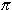# Practice Exercises: Circumference and AreaDirections: Read each question below. Click once in an ANSWER BOX and type in your answer; then click ENTER. After you click ENTER, a message will appear in the RESULTS BOX to indicate whether your answer is correct or incorrect. To start over, click CLEAR. Use= 3.14 to calculate your answers.

 1.If the diameter of a circle is 142.8 millimeters, then what is the radius? ANSWER BOX:=  mm  RESULTS BOX:
 2.If the radius of a circle is 15.3 centimeters, then what is the diameter? ANSWER BOX:=  cm  RESULTS BOX:
 3.Find the circumference of a circle with a diameter of 11 millimeters. ANSWER BOX:=  mm  RESULTS BOX:
 4.Find the circumference of a circle with a diameter of 7.5 inches. ANSWER BOX:=  in  RESULTS BOX:
 5.Find the diameter of a circle with a circumference of 21.98 meters. ANSWER BOX:=  m  RESULTS BOX:
 6.Find the area of a circle with a radius of 4 inches. ANSWER BOX:=  in2  RESULTS BOX:
 7.Find the area of a circle with a radius of 3.5 centimeters. (Do not round.  Enter your answer to three decimal places.) ANSWER BOX:= cm2  RESULTS BOX:
 8.Find the radius of a circle with an area of 200.96 square feet. ANSWER BOX:=  ft  RESULTS BOX:
 9.If the radius of a circle is 1.5 inches, then what is the circumference? ANSWER BOX:=  in  RESULTS BOX:
 10.If the diameter of a circle is 9 centimeters, then what is the area? (Do not round.  Enter your answer to three decimal places.) ANSWER BOX:=  cm2  RESULTS BOX:
 Circumference & Area Lessons Geometry and the Circle Circumference of a Circle Area of a Circle Practice Exercises Challenge Exercises Solutions